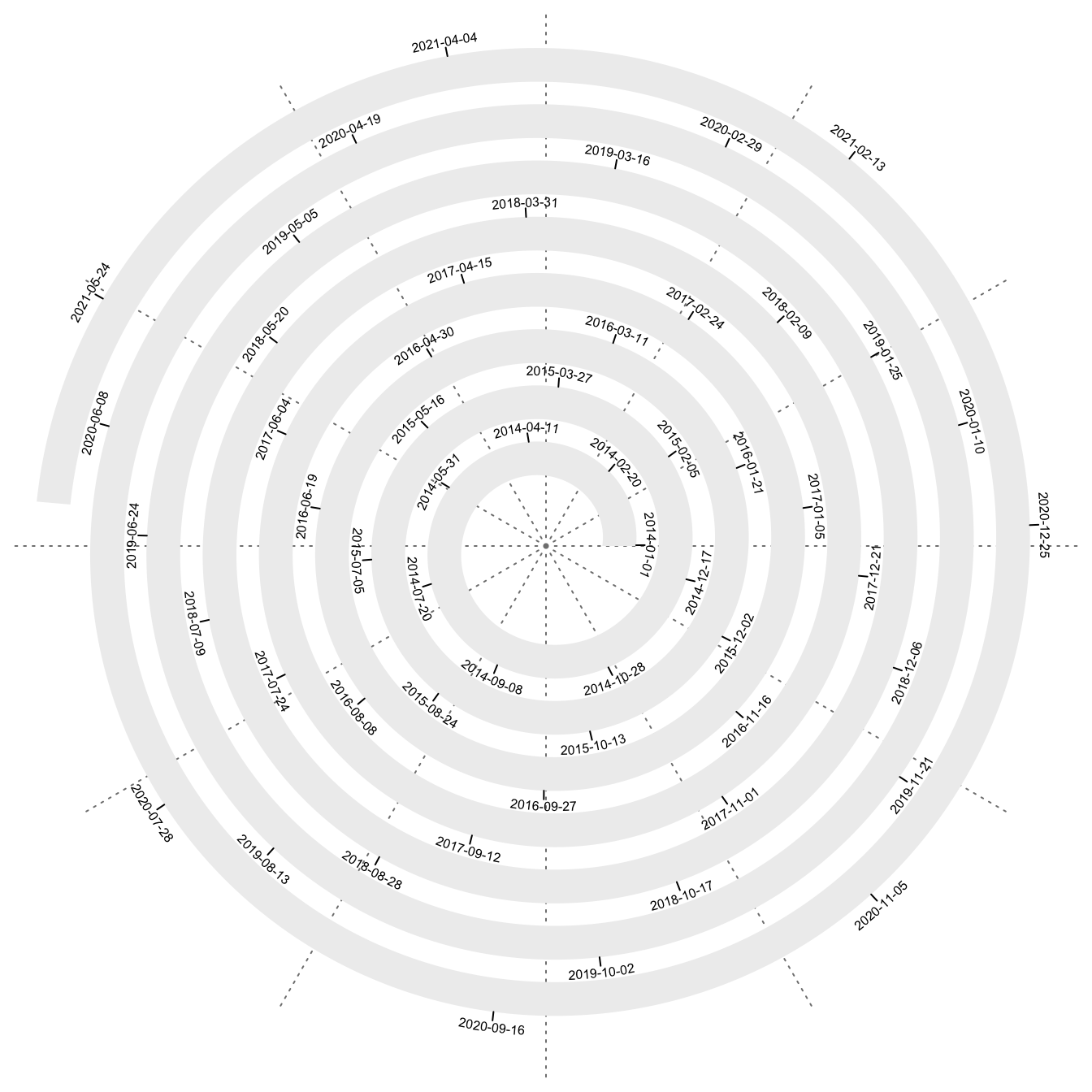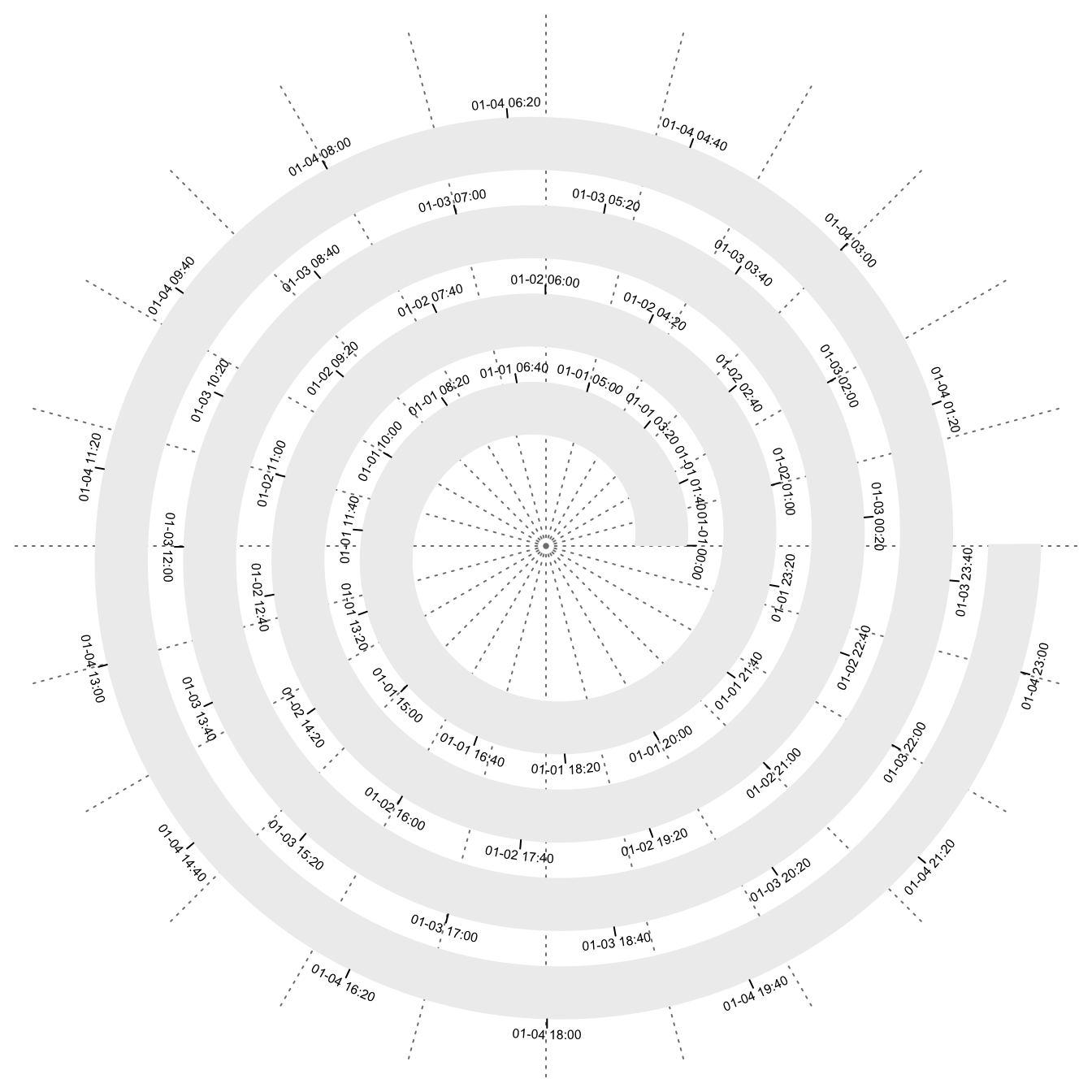# Initialize Spirals by Special Data Types

#### 2022-02-04

This vignette is built with spiralize 1.0.5.

## Work with date/time object

For time series data, values on x-axis are time points in a certain unit, e.g. days, or hours, and are linearly distributed along the axis. To map time series data to the spiral, internally a simple conversion is applied. Assume the first time point is `t1` and the last time point is `t2`, the number of time points is `n = t2 - t1` which is the time difference between the two. If we take `[0, 1]` for the first time point, `[1, 2]` for the second time point, and `[n-1, n]` for the last time point, then internally the spiral is initialized with `xlim = c(0, n)` and a time point `d` is converted to the internal numeric value by `d - d1 + 0.5`.

The unit of time point can be set via the argument `unit_on_axis` and the unit of the period can be set via the argument `period`, e.g. `unit_on_axis = "days"` and `period = "years"`. `unit_on_aixs` can be set as one of `"days"`, `"months"`, `"weeks"`, `"hours"`, `"mins"` and `"secs"`. And there are also corresponding values for `period`. If these two arguments are not set, they are guessed from `xlim` automatically.

In the following examples, also note the default value of `polar_lines_by` is also different for different `period`. E.g. there are 12 polar lines for years (12 months), 7 polar lines for weeks (7 weekdays), 24 polar lines for days (24 hours).

``spiral_initialize_by_time(xlim = c("2014-01-01", "2021-06-17"))``
``````## 'unit_to_axis' is set to 'days'.
## 'period' is set to 'years'.``````
``````## When the period is year and the unit on axis is day, a loop can only represent 364 days
## (52 weeks) under default settings, which helps to correspond weekdays between years, but
## 1 or 2 days from the current year will be moved and accumulated to the next year. You can
## set argument `normalize_year = TRUE` to enforce every loop to represent a complete year,
## but note you might not be able to perfectly correspond weekdays between different years.
## Set argument `verbose = FALSE` to turn off this message.``````
``````spiral_track(height = 0.6)
spiral_axis()````````spiral_initialize_by_time(xlim = c("2021-01-01 00:00:00", "2021-01-05 00:00:00"))``
``````## 'unit_to_axis' is set to 'mins'.
## 'period' is set to 'days'.``````
``````spiral_track(height = 0.6)
spiral_axis()````````````spiral_initialize_by_time(xlim = c("2021-01-01 00:00:00", "2021-01-01 00:10:00"),
unit_on_axis = "secs", period = "mins")
spiral_track(height = 0.6)
spiral_axis()``````